## Selina Concise Mathematics Class 10 ICSE Solutions Linear Inequations (in one variable)

Selina Publishers Concise Mathematics Class 10 ICSE Solutions Chapter 4 Linear Inequations (in one variable)

### Question 1.Solution: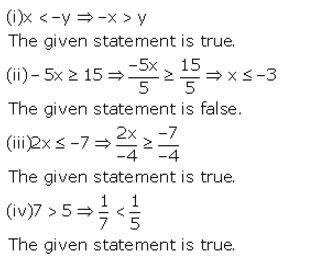Question 2.
State, whether the following statements are true or false:
(i) a < b, then a – c < b – c (ii) If a > b, then a + c > b + c
(iii) If a < b, then ac > bc
(iv) If a > b, then $$\frac { a }{ c } <\frac { b }{ c }$$
(v) If a – c > b – d, then a + d > b + c
(vi) If a < b, and c > 0, then a – c > b – c
Where a, b, c and d are real numbers and c ≠ 0.
Solution:
(i) a < b ⇒ a – c < b – c The given statement is true.
(ii) If a > b ⇒ a + c > b + c
The given statement is true.
(iii) If a < b ⇒ ac < bc The given statement is false.
(iv) If a > b ⇒ $$\frac { a }{ c } >\frac { b }{ c }$$
The given statement is false.
(v) If a – c > b – d ⇒ a + d > b + c
The given statement is true.
(vi) If a < b ⇒ a – c < b – c (Since, c > 0)
The given statement is false.

Question 3.
If x ∈ N, find the solution set of inequations.
(i) 5x + 3 ≤ 2x + 18
(ii) 3x – 2 < 19 – 4x
Solution:
(i) 5x + 3 ≤ 2x + 18
5x – 2x ≤ 18 – 3
3x ≤ 15
x ≤ 5
Since, x ∈ N, therefore solution set is {1, 2, 3, 4, 5}.
(ii) 3x – 2 < 19 – 4x
3x + 4x < 19 + 2
7x < 21
x < 3
Since, x ∈ N, therefore solution set is {1, 2}.

Question 4.Solution:
(i) x + 7 ≤ 11
x ≤ 11 – 7
x ≤ 4
Since, the replacement set = W (set of whole numbers)
⇒ Solution set = {0, 1, 2, 3, 4}
(ii) 3x – 1 > 8
3x > 8 + 1
x > 3
Since, the replacement set = W (set of whole numbers)
⇒ Solution set = {4, 5, 6, …}
(iii) 8 – x > 5
– x > 5 – 8
– x > -3
x < 3
Since, the replacement set = W (set of whole numbers)
⇒ Solution set = {0, 1, 2}Since, the replacement set = W (set of whole numbers)
∴ Solution set = {0, 1, 2}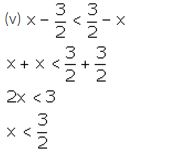Since, the replacement set = W (set of whole numbers)
∴ Solution set = {0, 1}
(vi) 18 ≤ 3x – 2
18 + 2 ≤ 3x
20 ≤ 3x
x ≥ $$\frac { 20 }{ 3 }$$
Since, the replacement set = W (set of whole numbers)
∴ Solution set = {7, 8, 9, …}

Question 5.
Solve the inequation:
3 – 2x ≥ x – 12 given that x ∈ N.
Solution:
3 – 2x ≥ x – 12
-2x – x ≥ -12 – 3
-3x ≥ -15
x ≤ 5
Since, x ∈ N, therefore,
Solution set = {1, 2, 3, 4, 5}

Question 6.
If 25 – 4x ≤ 16, find:
(i) the smallest value of x, when x is a real number,
(ii) the smallest value of x, when x is an integer.
Solution:
25 – 4x ≤ 16
-4x ≤ 16 – 25
-4x ≤ -9
x ≥ $$\frac { 9 }{ 4 }$$
x ≥ 2.25
(i) The smallest value of x, when x is a real number, is 2.25.
(ii) The smallest value of x, when x is an integer, is 3.

Question 7.Solution: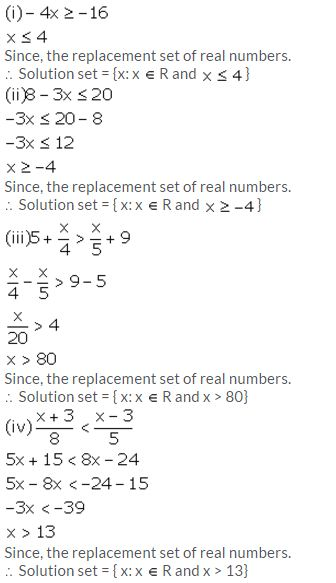Question 8.Solution:Thus, the required smallest value of x is -1.

Question 9.
Find the largest value of x for which
2(x – 1) ≤ 9 – x and x ∈ W.
Solution:
2(x – 1) ≤ 9 – x
2x – 2 ≤ 9 – x
2x + x ≤ 9 + 2
3x ≤ 11
x ≤ $$\frac { 11 }{ 3 }$$
x ≤ 3.67
Since, x ∈ W, thus the required largest value of x is 3.

Question 10.Solution:Question 11.
Given x ∈ {integers}, find the solution set of:
-5 ≤ 2x – 3 < x + 2
Solution: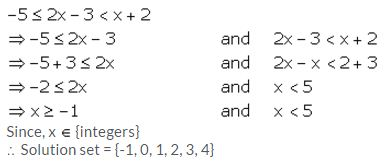Question 12.
Given x ∈ {whole numbers}, find the solution set of:
-1 ≤ 3 + 4x < 23

Solution:### Linear Inequations in One Variable Exercise 4B – Selina Concise Mathematics Class 10 ICSE Solutions

Question 1.Solution: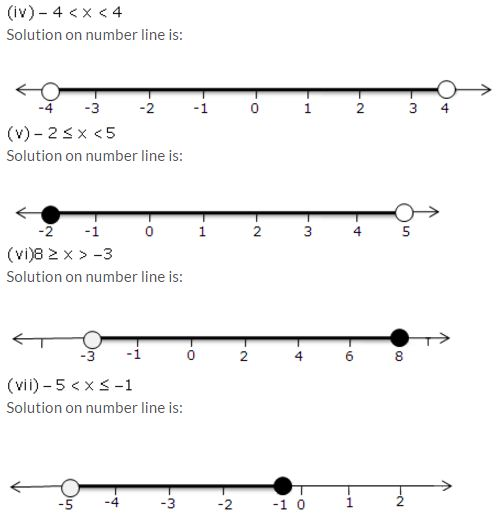Question 2.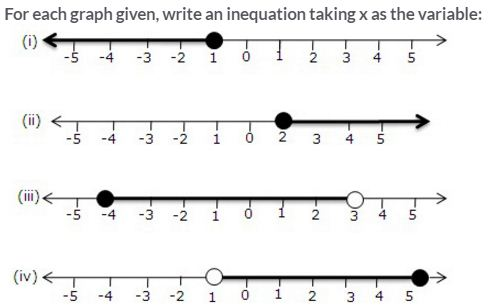Solution:Question 3.Solution:Question 4.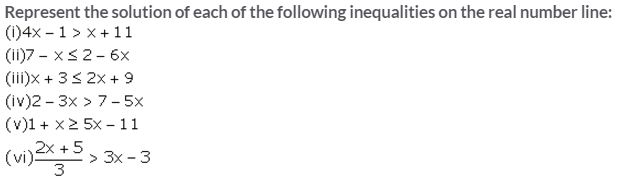Solution: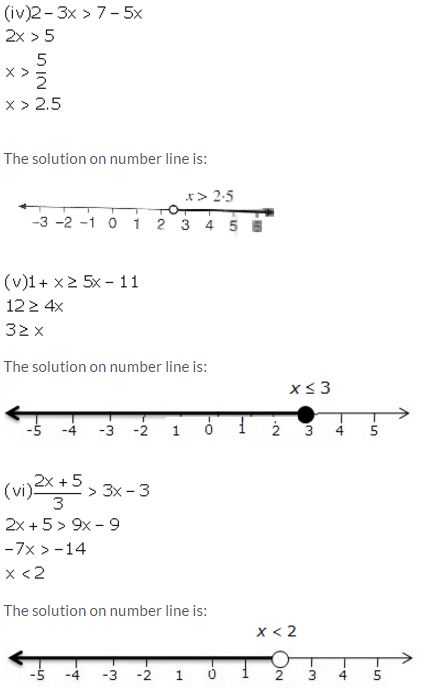Question 5.
x ∈ {real numbers} and -1 < 3 – 2x ≤ 7, evaluate x and represent it on a number line.
Solution:
-1 < 3 – 2x ≤ 7
-1 < 3 – 2x and 3 – 2x ≤ 7
2x < 4 and -2x ≤ 4
x < 2 and x ≥ -2
Solution set = {-2 ≤ x < 2, x ∈ R}
Thus, the solution can be represented on a number line as:Question 6.
List the elements of the solution set of the inequation
-3 < x – 2 ≤ 9 – 2x; x ∈ N.
Solution:
-3 < x – 2 ≤ 9 – 2x
-3 < x – 2 and x – 2 ≤ 9 – 2x
-1 < x and 3x ≤ 11
-1 < x ≤ $$\frac { 11 }{ 3 }$$
Since, x ∈ N
∴ Solution set = {1, 2, 3}

Question 7.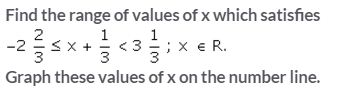Solution: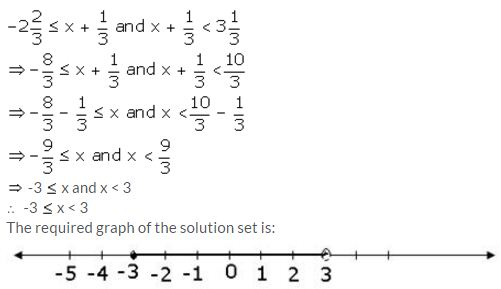Question 8.Solution: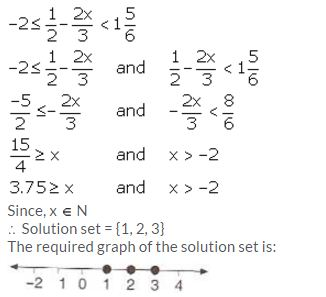Question 9.
Given x ∈ {real numbers}, find the range of values of x for which -5 ≤ 2x – 3 < x + 2 and represent it on a number line.
Solution:
-5 ≤ 2x – 3 < x + 2
-5 ≤ 2x – 3 and 2x – 3 < x + 2
-2 ≤ 2x and x < 5
-1 ≤ x and x < 5
Required range is -1 ≤ x < 5.
The required graph is: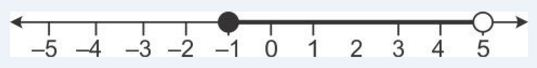Question 10.
If 5x – 3 ≤ 5 + 3x ≤ 4x + 2, express it as a ≤ x ≤ b and then state the values of a and b.
Solution:
5x – 3 ≤ 5 + 3x ≤ 4x + 2
5x – 3 ≤ 5 + 3x and 5 + 3x ≤ 4x + 2
2x ≤ 8 and -x ≤ -3
x ≤ 4 and x ≥ 3
Thus, 3 ≤  x ≤ 4.
Hence, a = 3 and b = 4.

Question 11.
Solve the following inequation and graph the solution set on the number line:
2x – 3 < x + 2 ≤ 3x + 5, x ∈ R.
Solution:
2x – 3 < x + 2 ≤ 3x + 5
2x – 3 < x + 2 and x + 2 ≤ 3x + 5
x < 5 and -3 ≤ 2x
x < 5 and -1.5 ≤ x
Solution set = {-1.5 ≤ x < 5}
The solution set can be graphed on the number line as: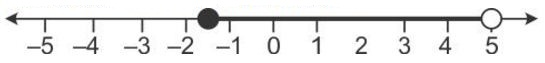Question 12.
Solve and graph the solution set of:
(i) 2x – 9 < 7 and 3x + 9 ≤ 25, x ∈ R (ii) 2x – 9 ≤ 7 and 3x + 9 > 25, x ∈ I
(iii) x + 5 ≥ 4(x – 1) and 3 – 2x < -7, x ∈ R
Solution:Question 13.
Solve and graph the solution set of:
(i) 3x – 2 > 19 or 3 – 2x ≥ -7, x ∈ R
(ii) 5 > p – 1 > 2 or 7 ≤ 2p – 1 ≤ 17, p ∈ R
Solution:
(i) 3x – 2 > 19 or 3 – 2x ≥ -7
3x > 21 or -2x ≥ -10
x > 7 or x ≤ 5
Graph of solution set of x > 7 or x ≤ 5 = Graph of points which belong to x > 7 or x ≤ 5 or both.
Thus, the graph of the solution set is: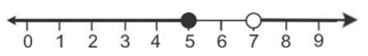(ii) 5 > p – 1 > 2 or 7 ≤ 2p – 1 ≤ 17
6 > p > 3 or 8 ≤ 2p ≤ 18
6 > p > 3 or 4 ≤ p ≤ 9
Graph of solution set of 6 > p > 3 or 4 ≤ p ≤ 9
= Graph of points which belong to 6 > p > 3 or 4 ≤ p ≤ 9 or both
= Graph of points which belong to 3 < p ≤ 9
Thus, the graph of the solution set is: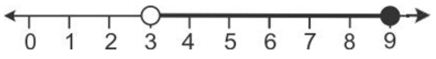Question 14.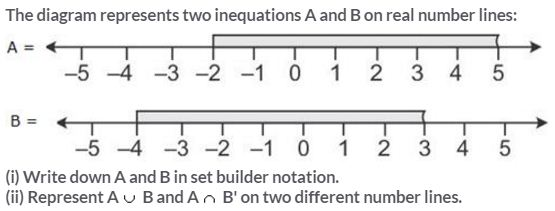Solution:
(i) A = {x ∈ R: -2 ≤ x < 5}
B = {x ∈ R: -4 ≤ x < 3}
(ii) A ∩ B = {x ∈ R: -2 ≤ x < 5}
It can be represented on number line as:B’ = {x ∈ R: 3 < x ≤ -4}
A ∩ B’ = {x ∈ R: 3 ≤ x < 5}
It can be represented on number line as: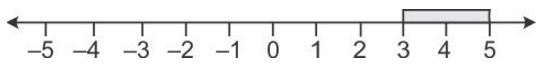Question 15.
Use real number line to find the range of values of x for which:
(i) x > 3 and 0 < x < 6
(ii) x < 0 and -3 ≤ x < 1
(iii) -1 < x ≤ 6 and -2 ≤ x ≤ 3
Solution:
(i) x > 3 and 0 < x < 6
Both the given inequations are true in the range where their graphs on the real number lines overlap.
The graphs of the given inequations can be drawn as: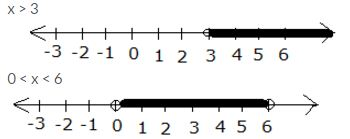From both graphs, it is clear that their common range is
3 < x < 6
(ii) x < 0 and -3 ≤ x < 1
Both the given inequations are true in the range where their graphs on the real number lines overlap.
The graphs of the given inequations can be drawn as:From both graphs, it is clear that their common range is
-3 ≤ x < 0
(iii) -1 < x ≤ 6 and -2 ≤ x ≤ 3
Both the given inequations are true in the range where their graphs on the real number lines overlap.
The graphs of the given inequations can be drawn as: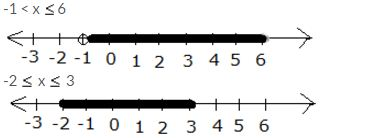From both graphs, it is clear that their common range is
-1 < x ≤ 3

Question 16.
Illustrate the set {x: -3 ≤ x < 0 or x > 2, x ∈ R} on the real number line.
Solution:
Graph of solution set of -3 ≤ x < 0 or x > 2
= Graph of points which belong to -3 ≤ x < 0 or x > 2 or both
Thus, the required graph is: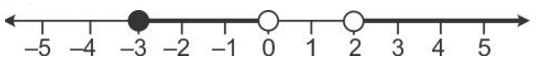Question 17.
Given A = {x: -1 < x ≤ 5, x ∈ R} and B = {x: -4 ≤ x < 3, x ∈ R}
Represent on different number lines:
(i) A ∩ B
(ii) A’ ∩ B
(iii) A – B
Solution: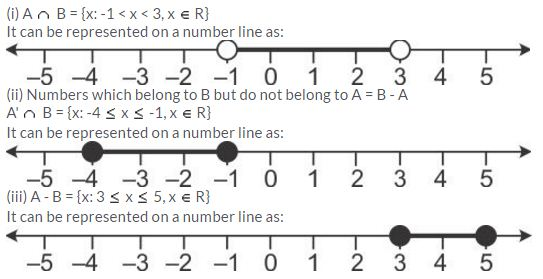Question 18.
P is the solution set of 7x – 2 > 4x + 1 and Q is the solution set of 9x – 45 ≥ 5(x – 5); where x ∈ R. Represent:
(i) P ∩ Q
(ii) P – Q
(iii) P ∩ Q’
on different number lines.
Solution: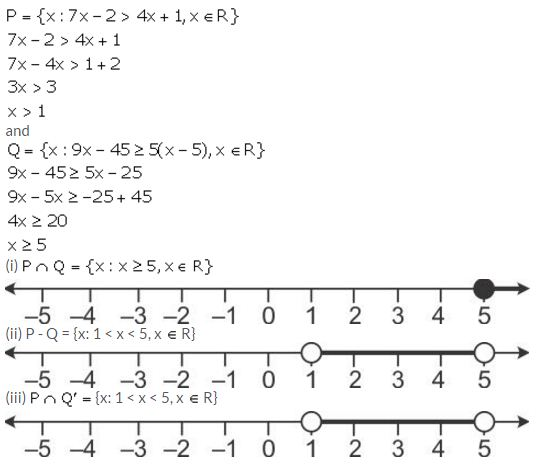Question 19.Solution: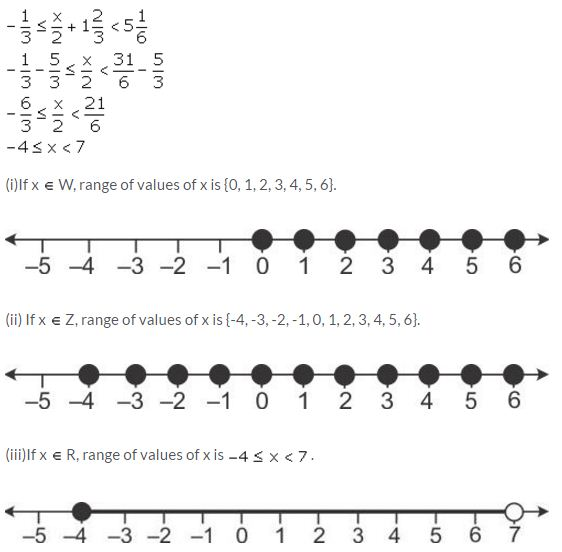Question 20.
Given: A = {x: -8 < 5x + 2 ≤ 17, x ∈ I}, B = {x: -2 ≤ 7 + 3x < 17, x ∈ R}
Where R = {real numbers} and I = {integers}. Represent A and B on two different number lines. Write down the elements of A ∩ B.
Solution:Question 21.
Solve the following inequation and represent the solution set on the number line 2x – 5 ≤ 5x +4 < 11, where x ∈ I
Solution: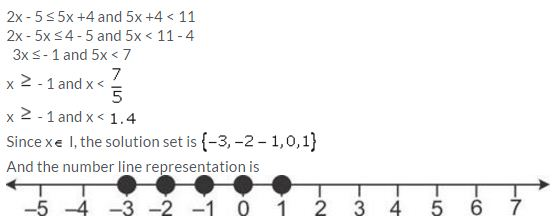Question 22.Solution: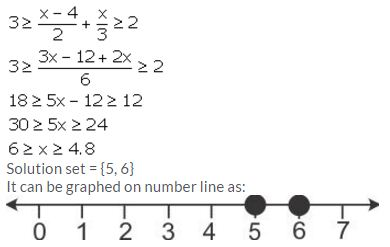Question 23.
Given:
A = {x: 11x – 5 > 7x + 3, x ∈ R} and
B = {x: 18x – 9 ≥ 15 + 12x, x ∈ R}.
Find the range of set A ∩ B and represent it on number line.
Solution:
A = {x: 11x – 5 > 7x + 3, x ∈ R}
= {x: 4x > 8, x ∈ R}
= {x: x > 2, x ∈ R}
B = {x: 18x – 9 ≥ 15 + 12x, x ∈ R}
= {x: 6x ≥ 24, x ∈ R}
= {x: x ≥ 4, x ∈ R}
A ∩ B = {x: x ≥ 4, x ∈ R}
It can be represented on number line as:Question 24.Solution:Question 25.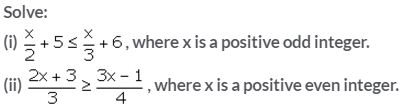Solution:Question 26.Solution: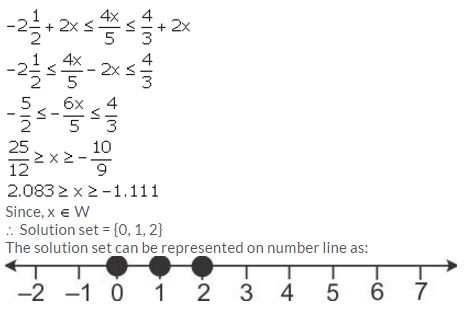Question 27.
Find three consecutive largest positive integers such that the sum of one-third of first, one-fourth of second and one-fifth of third is atmost 20.
Solution:
Let the required integers be x, x + 1 and x + 2.
According to the given statement,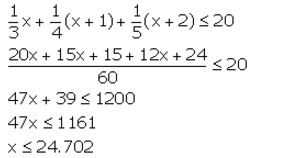Thus, the largest value of the positive integer x is 24.
Hence, the required integers are 24, 25 and 26.

Question 28.
Solve the given inequation and graph the solution on the number line.
2y – 3 < y + 1 ≤ 4y + 7, y ∈ R
Solution:
2y – 3 < y + 1 ≤ 4y + 7, y ∈ R
⇒ 2y – 3 – y < y + 1 – y ≤ 4y + 7 – y
⇒ y – 3 < 1 ≤ 3y + 7
⇒ y – 3 < 1 and 1 ≤ 3y + 7
⇒ y < 4 and 3y ≥ 6 ⇒ y ≥ – 2
⇒ – 2 ≤ y < 4
The graph of the given equation can be represented on a number line as:Question 29.
Solve the inequation:
3z – 5 ≤ z + 3 < 5z – 9, z ∈ R.
Graph the solution set on the number line.
Solution:
3z – 5 ≤ z + 3 < 5z – 9
3z – 5 ≤ z + 3 and z + 3 < 5z – 9
2z ≤ 8 and 12 < 4z
z ≤ 4 and 3 < z
Since, z R
∴ Solution set = {3 < z ≤ 4, x ∈ R }
It can be represented on a number line as:Question 30.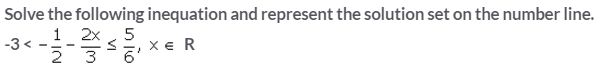Solution:Question 31.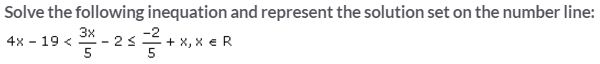Solution: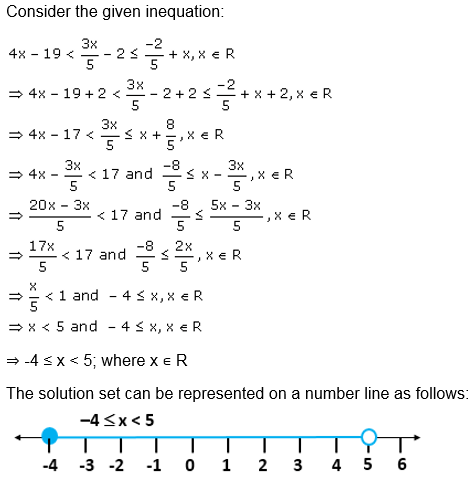Question 32.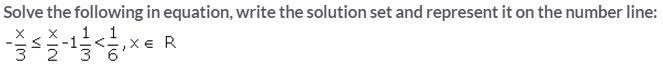Solution: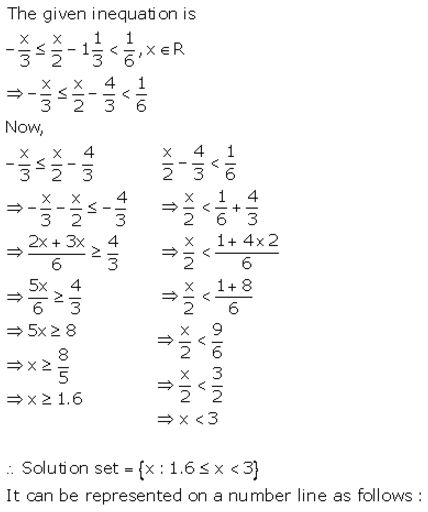Question 33.Solution: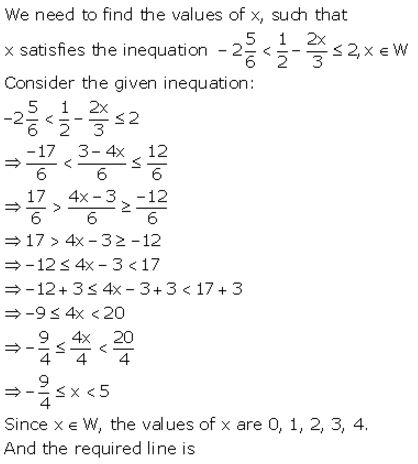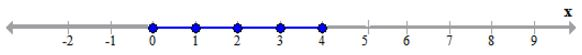Question 34.
Solve the following in equation and write the solution set:
13x – 5 < 15x + 4 < 7x + 12, x ∈ R
Solution: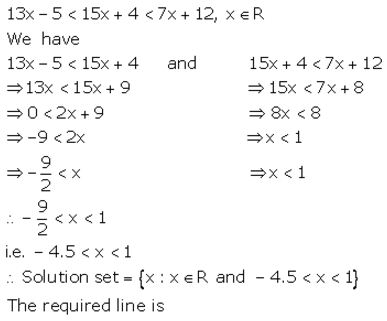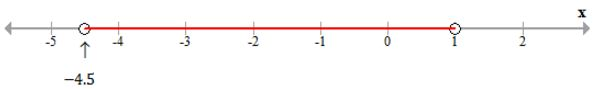Question 35.
Solve the following inequation, write the solution set and represent it on the number line.
-3(x – 7) ≥ 15 – 7x > x+1/3, x R.
Solution: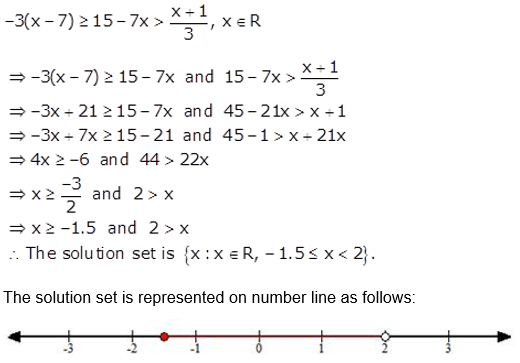Question 36.
Solve the following inequation and represent the solution set on a number line.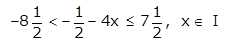Solution:More Resources for Selina Concise Class 10 ICSE Solutions